Home > CC4 > Chapter A > Lesson A.1.7 > ProblemA-76

A-76.

Rewrite each expression below as an equivalent expression without parentheses or negative exponents. Assume that the denominator in part (b) is not equal to zero. Homework Help ✎

1. (x3)(x−2)

2.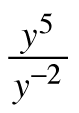3. 4−1

4. (4x2)3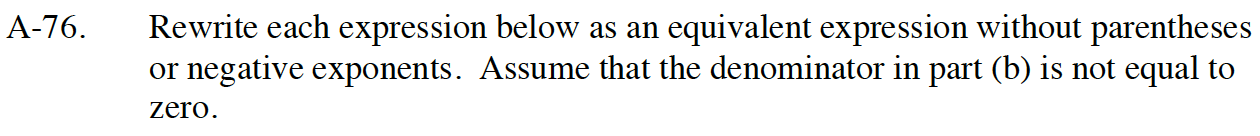Read Math Note box 1.3.2 on rules of exponents.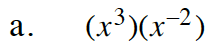When multiplying exponents with the same base, add the exponents.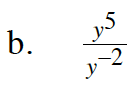When dividing exponents with the same base, subtract the denominator exponent from the numerator exponent. Notice that in this case you are subtracting a negative number: 5 − (−2).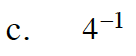4−1 is the reciprocal of 4.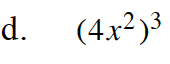To raise a power to a power, multiply the exponents like so: 41·3x2·3